# RS Aggarwal Solutions for Class 9 Chapter 1: Number Systems Exercise 1B

## RS Aggarwal Solutions for Class 9 Maths Exercise 1B PDF

Exercise 1B in the RS Aggarwal Solutions for Class 9 Maths provides accurate solutions to problems based on the evaluation method used in the exams. This exercise mainly explains in brief about the decimal representation of rational numbers. The solutions are explained in simple language keeping in mind the understanding ability of the students. Our set of subject experts work hard to provide the solutions solved in different methods as per the RS Aggarwal textbook from the exam point of view. RS Aggarwal Solutions for Class 9 Maths Chapter 1 Number Systems Exercise 1B are provided here.

## RS Aggarwal Solutions for Class 9 Chapter 1: Number Systems Exercise 1B Download PDF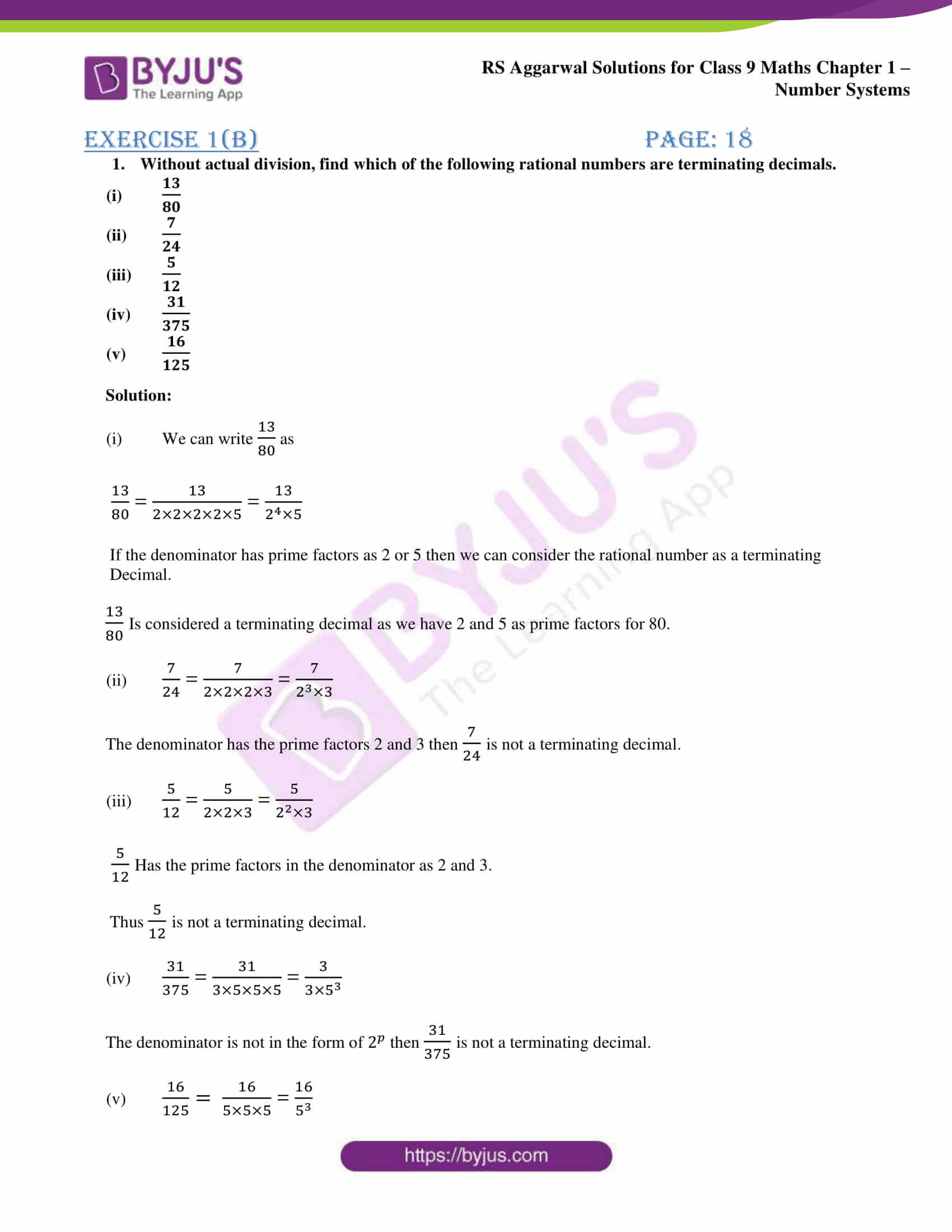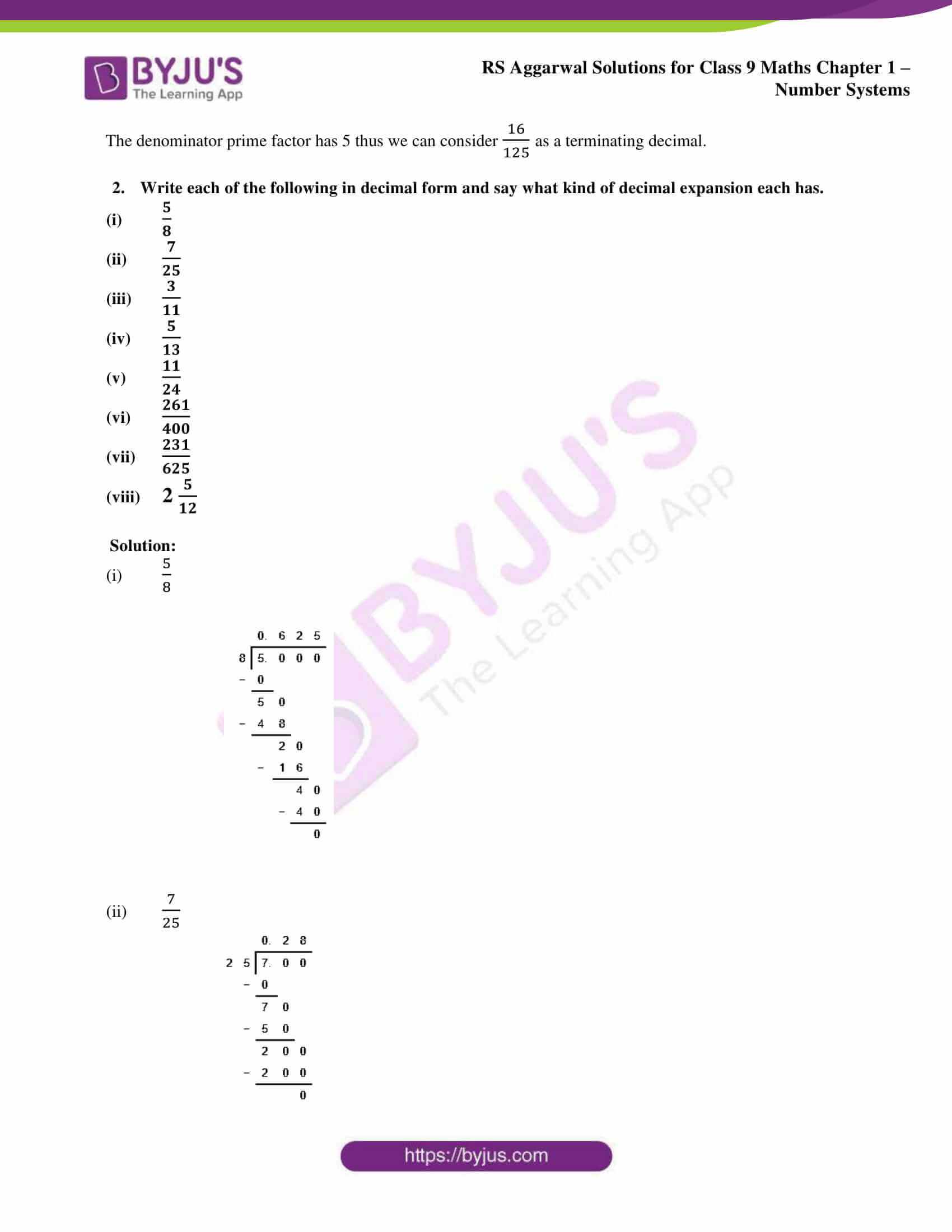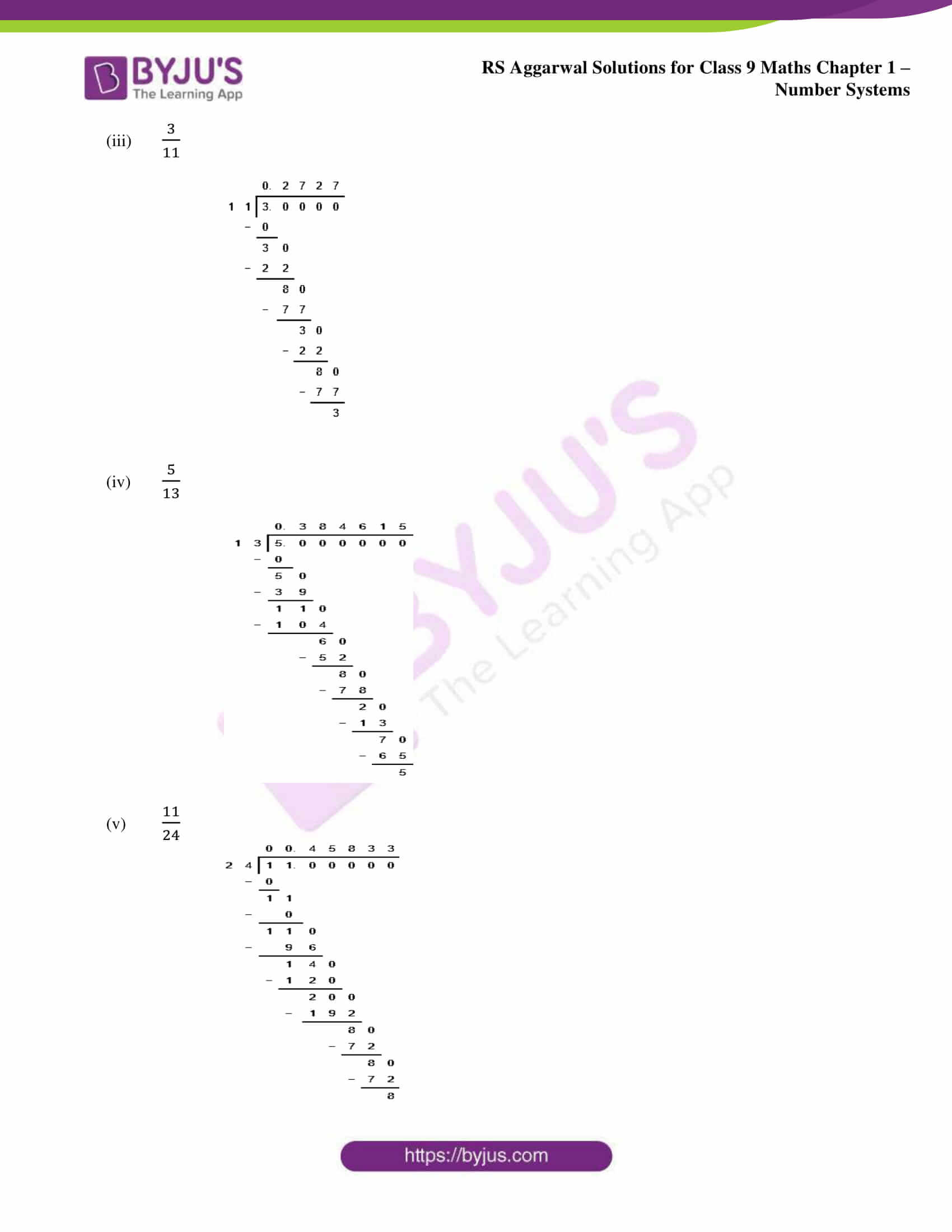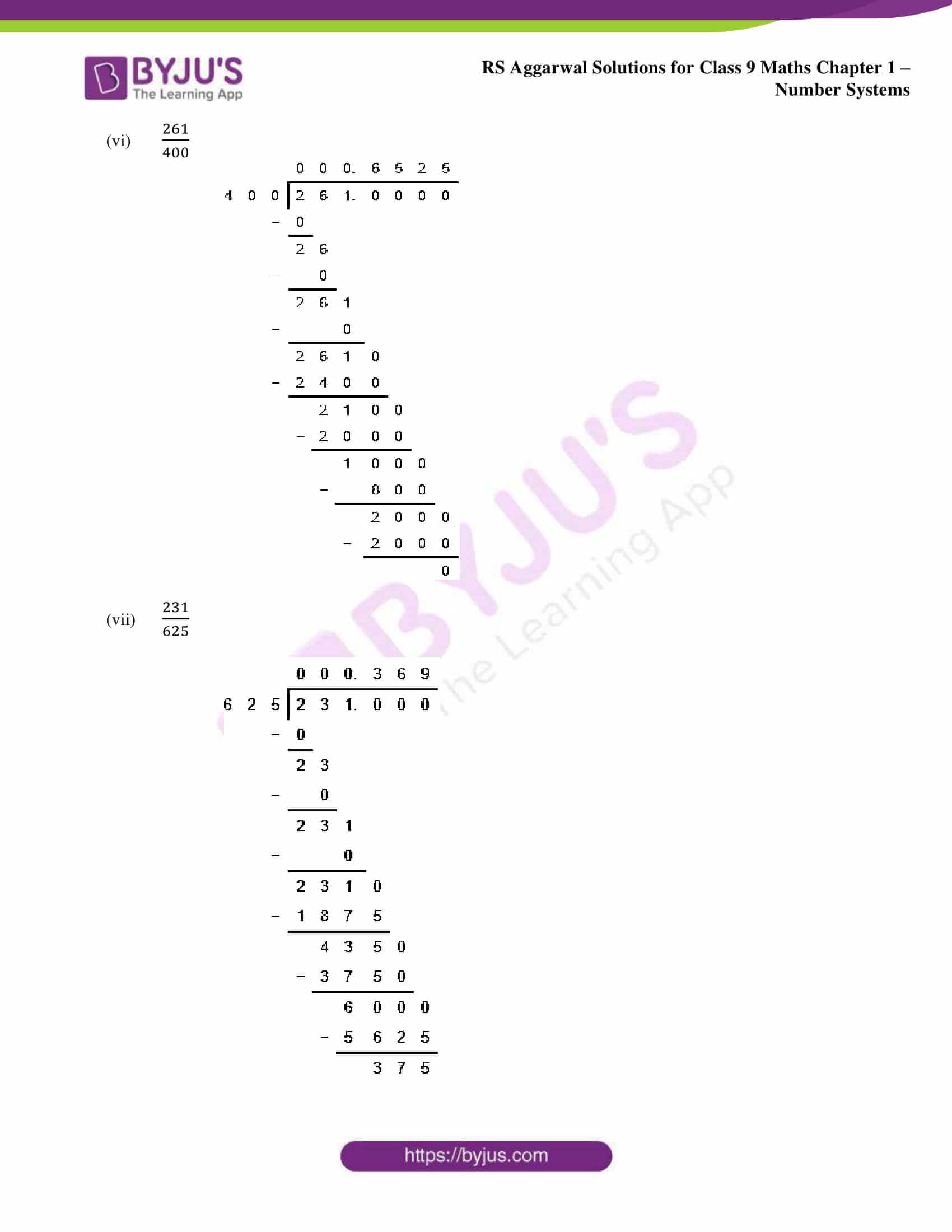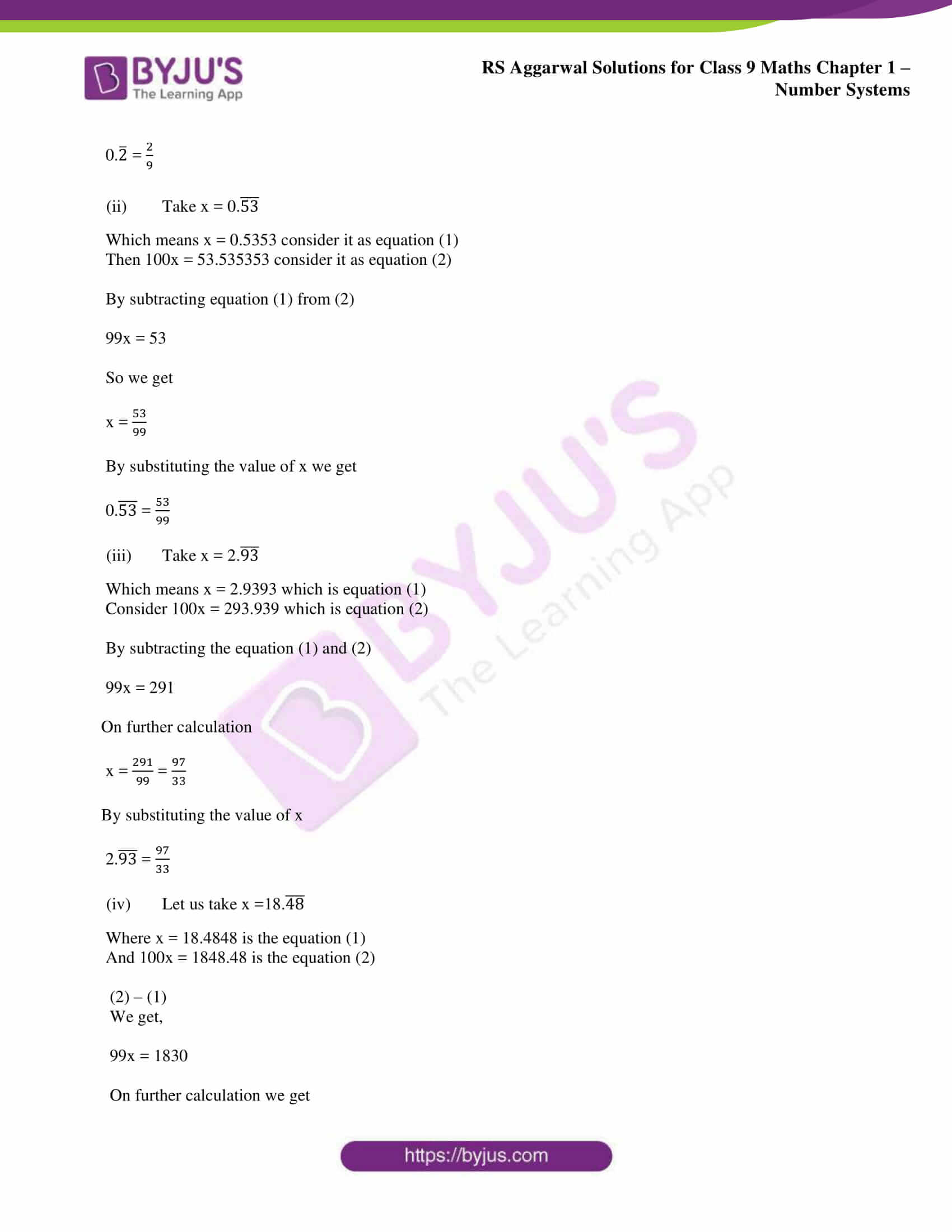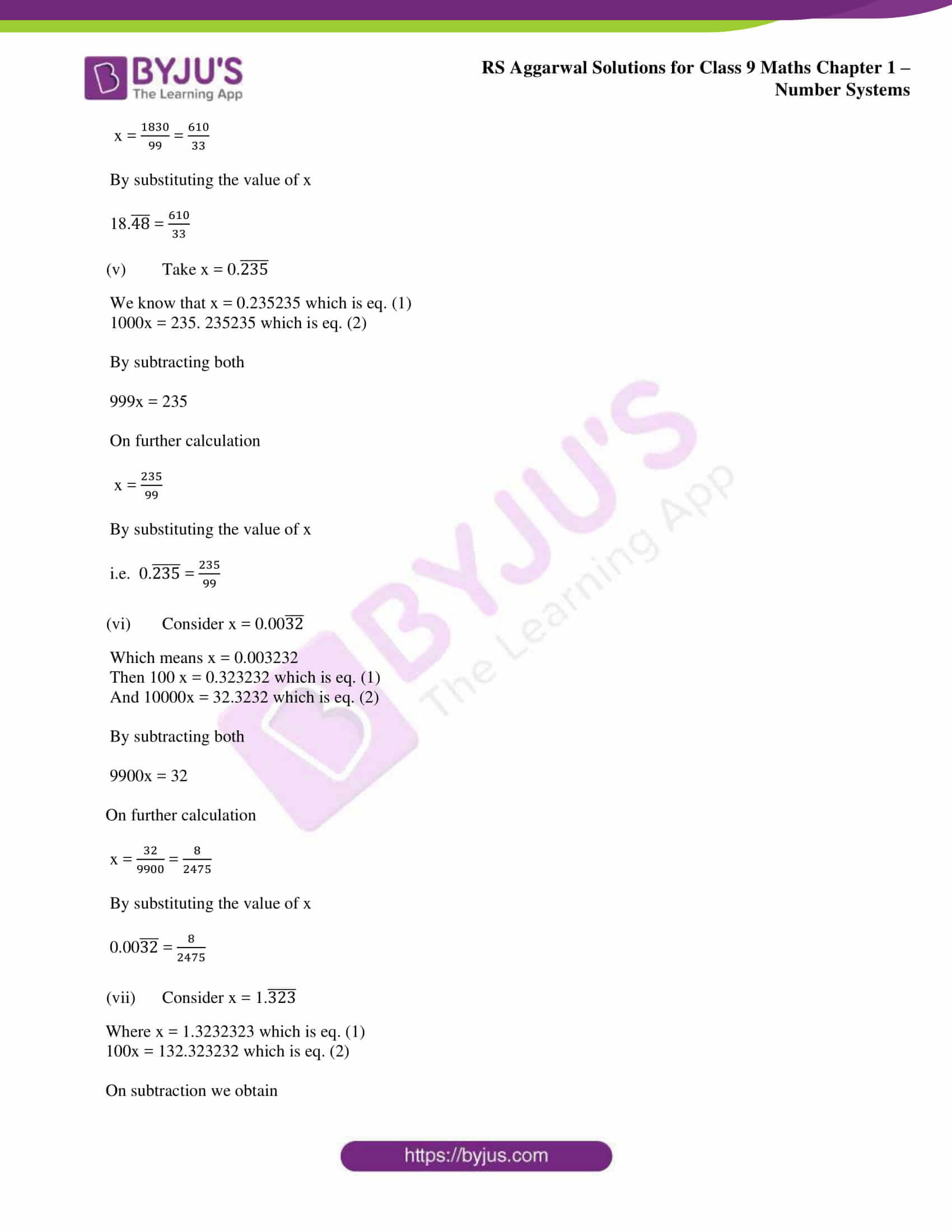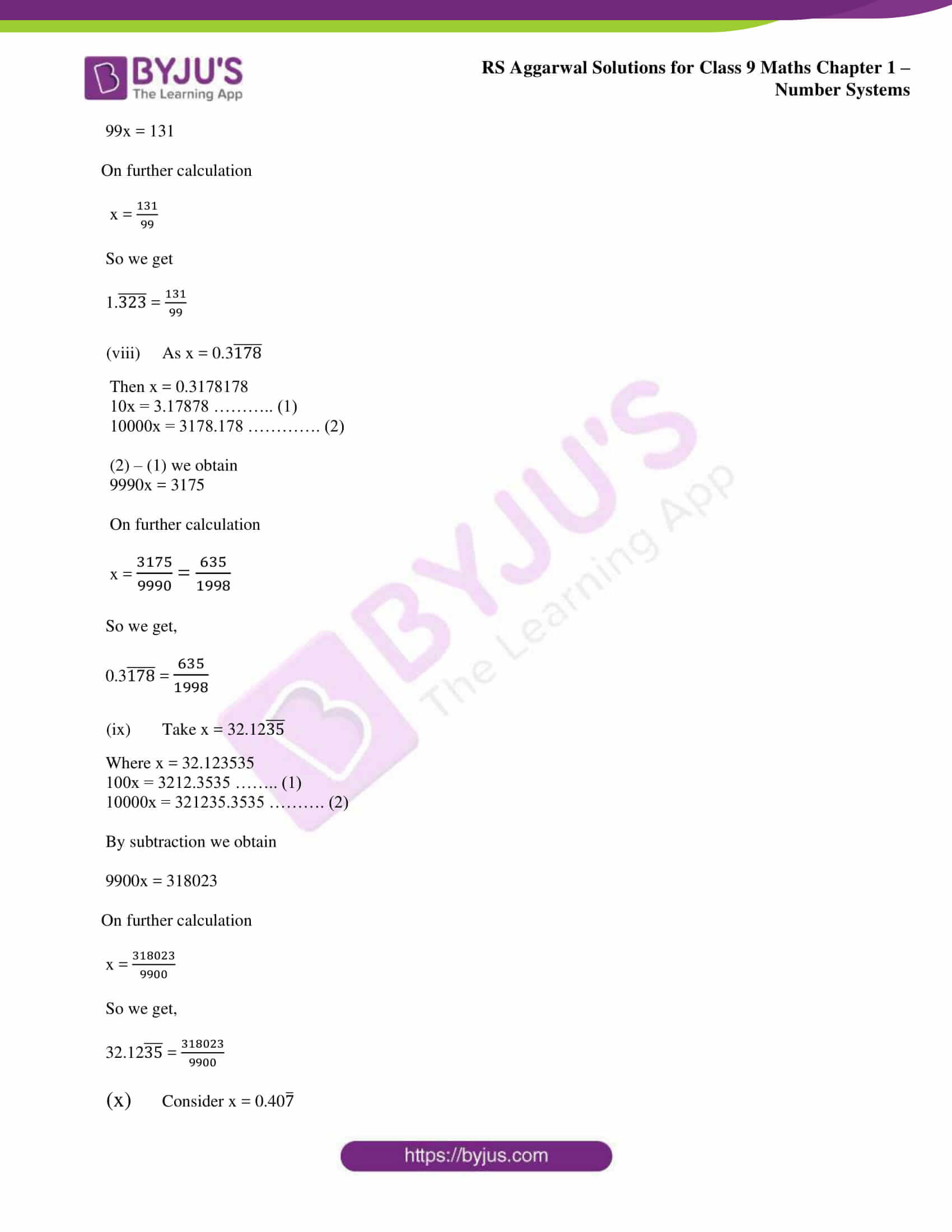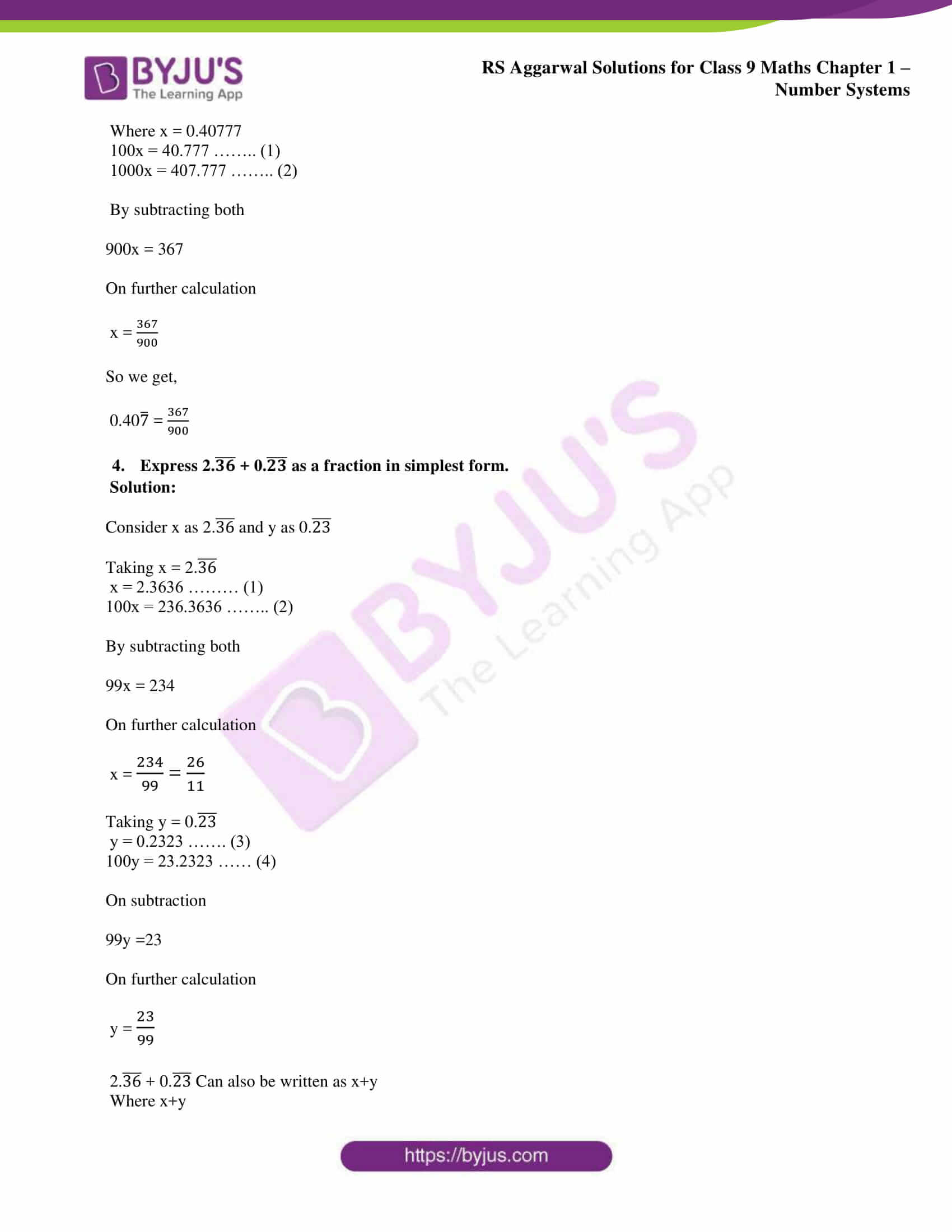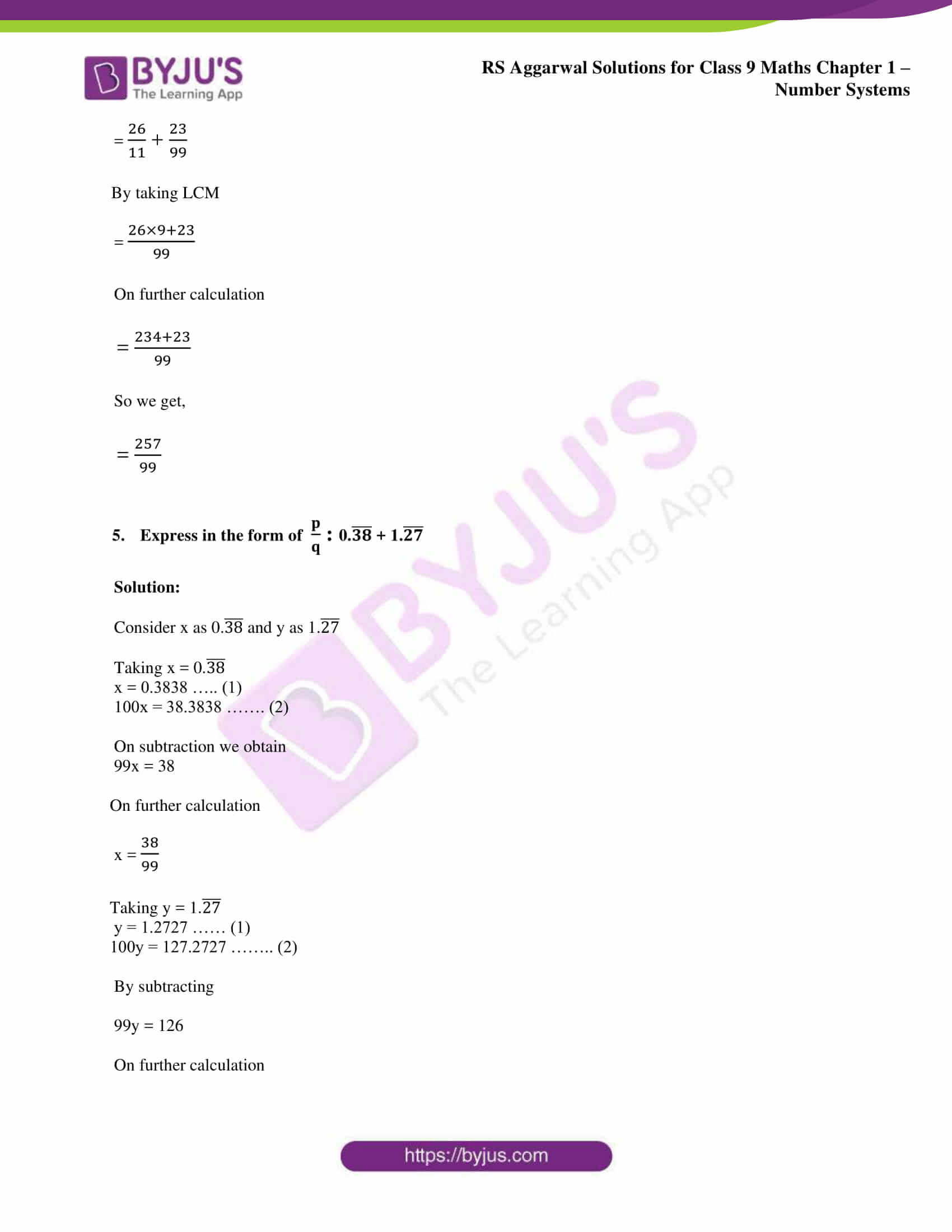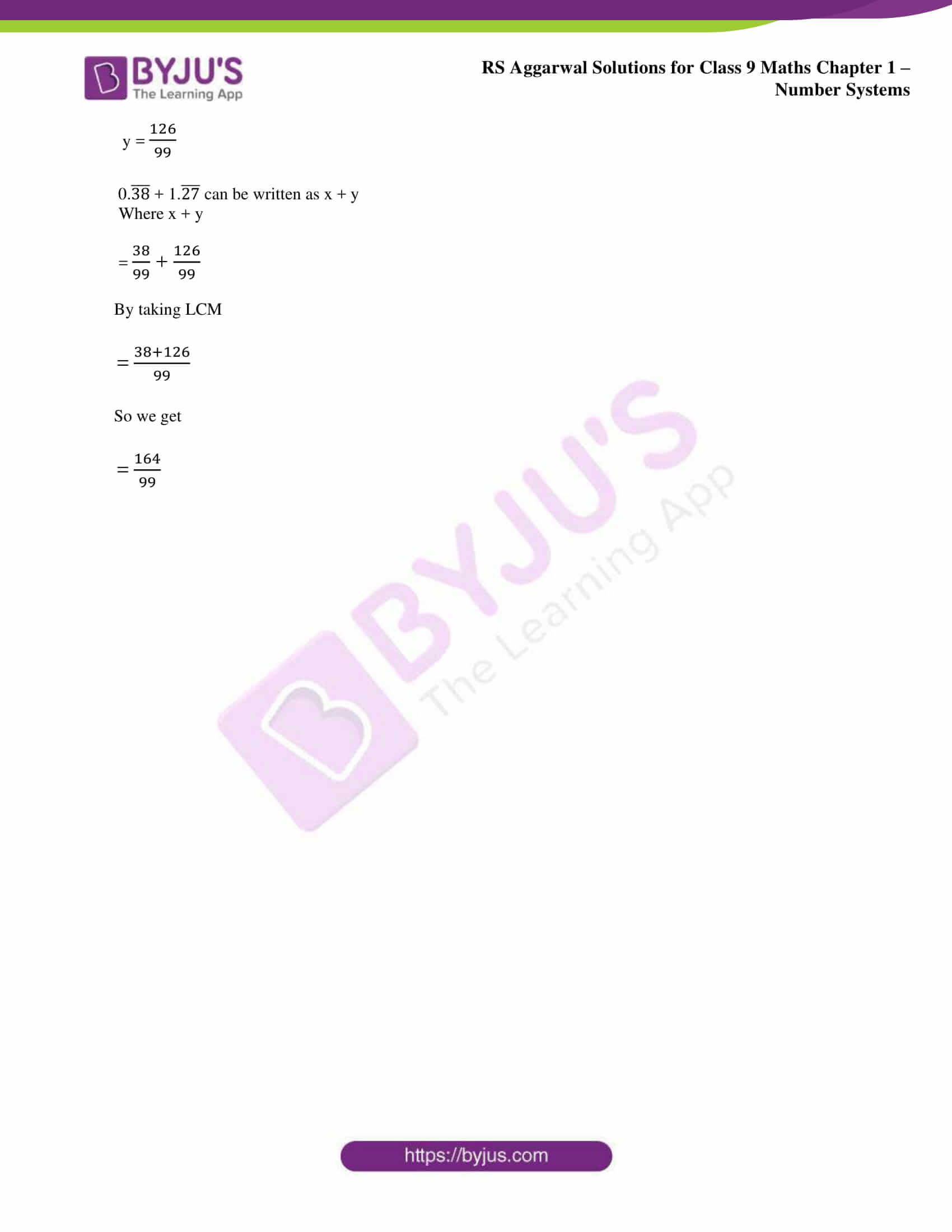### Access other exercise solutions of class 9 Maths Chapter 1: Number Systems

Exercise 1A Solutions 9 Questions

Exercise 1C Solutions 14 Questions

Exercise 1D Solutions 8 Questions

Exercise 1E Solutions 10 Questions

Exercise 1F Solutions 25 Questions

Exercise 1G Solutions 18 Questions

### RS Aggarwal Solutions Class 9 Maths Chapter 1 – Number Systems Exercise 1B

RS Aggarwal Solutions Class 9 Maths Chapter 1 Number Systems Exercise 1B provides step by step solutions using different ways for the decimal representation of rational numbers.

### Key features of RS Aggarwal Solutions for Class 9 Maths Chapter 1: Number Systems Exercise 1B

• The subject experts provide solutions to the exercises based on the RS Aggarwal guidelines.
• PDF material is available for each exercise to improve the problem solving ability among the students.
• The solutions are based on the evaluation, which is followed in the class 9 exams to help students with a chance of better academic performance.
• Each exercise is provided with more number of questions in order to make the students familiar with the concepts.